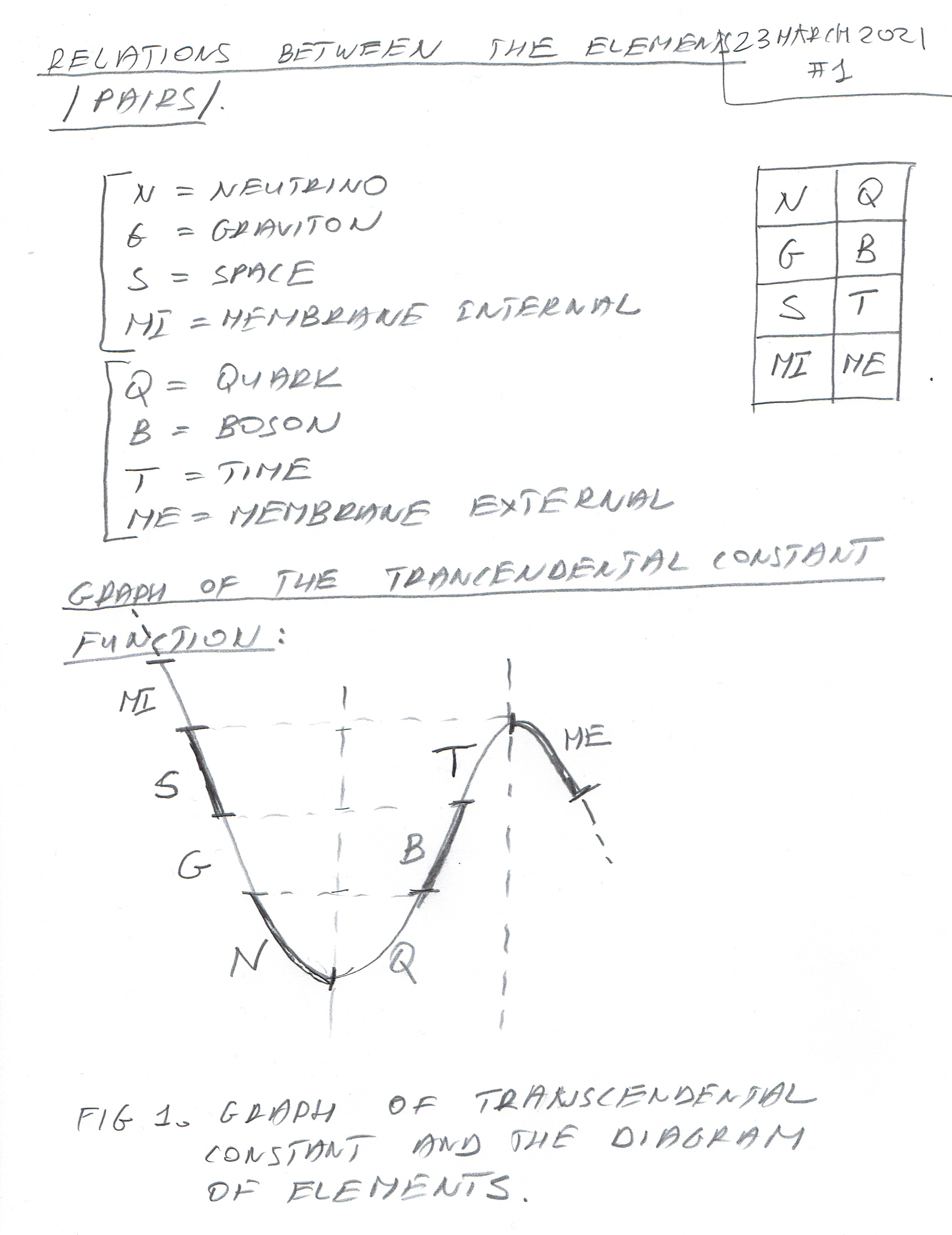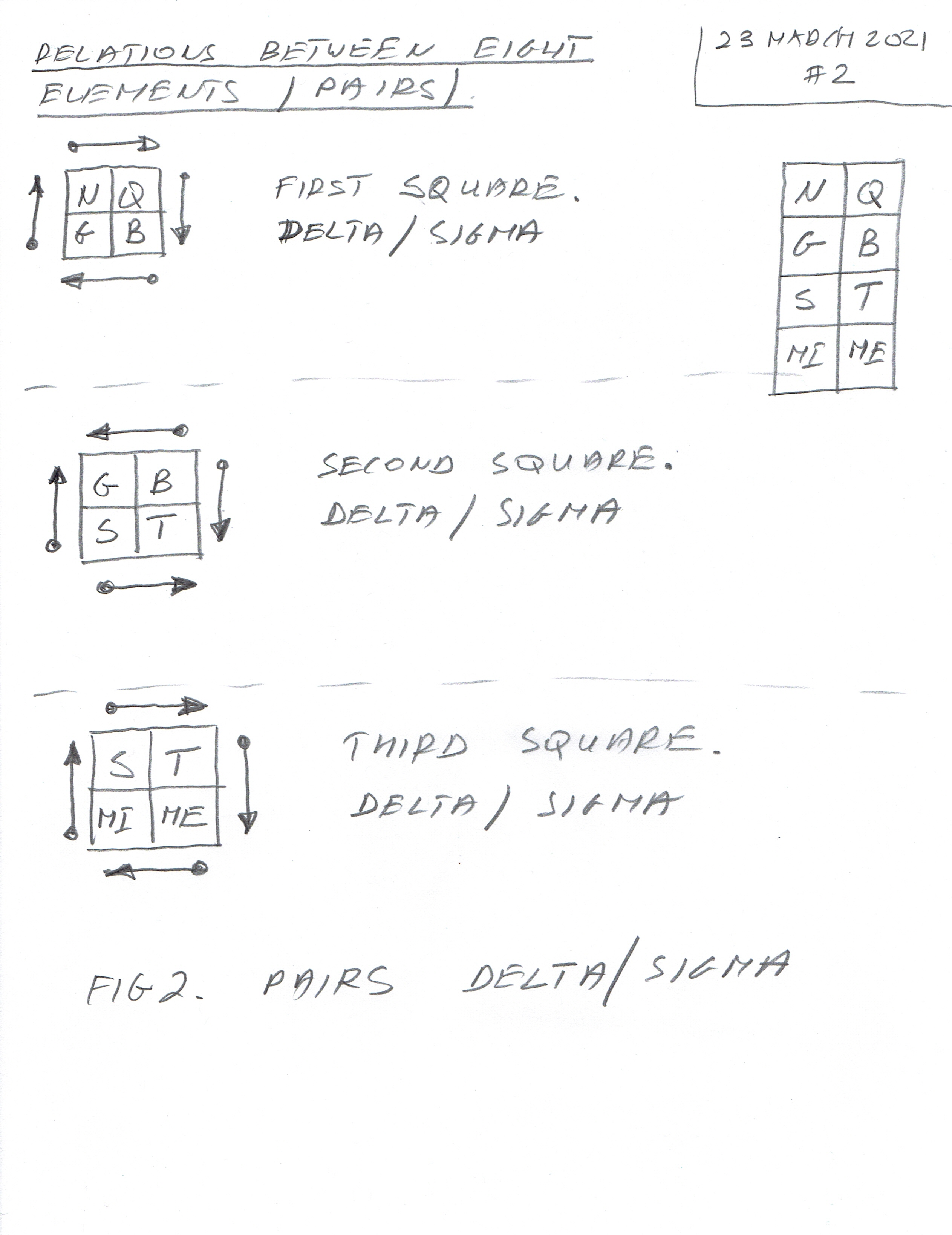This article deals with pairs selected from eight elements of the Universe - Neutrino, Graviton, Space, Membrane Internal and Quark, Boson, Time, Membrane External.

In Figure 1. you can see the graph of the Transcendental Constant Function and its representation in Diagram. The eight elements give us three squares with four elements each, as shown in Figure 2. The arrows show the direction of the interaction between the Delta CP Violating Phase Angles and Sigma Angles /Sums of three thetas mixing (oscillation) angles from before and after transform/. The Sigma Transform is just the folding of the Negative Real part of the complex plane onto the positive part of the Real Plane.

So, we have three squares with four elements each - the first one is Neutrino, Graviton, Quark, Boson; the second one is: Graviton, Space, Boson, Time; and the third one is: Space, Membrane Internal, Time, Membrane External. These pairs formed by the four elements in each show relations between Delta and Sigma Angles (more in the main articles - I will show the simple relations between those angles as well as the calculations of those angles).

Figure 1 - The Graph of the Transcendental Function and the Diagram of Elements.Figure 2 - Pairs of the Elements with Delta/Sigma Angles relations.